# Sugars

In what ratio must two sorts of sugar, costing #390 and #315 per kg respectively, be mixed in order to produce a mixture worth #369 per kg?

r =  18:7

### Step-by-step explanation: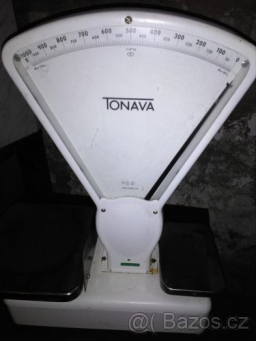Did you find an error or inaccuracy? Feel free to write us. Thank you!Tips to related online calculators
Check out our ratio calculator.
Do you have a linear equation or system of equations and looking for its solution? Or do you have a quadratic equation?

## Related math problems and questions:

• Coffee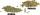Coffee merchant has coffee robusta and arabica species. 1 kg Robusta worth 450 CZK, Arabica 1 kg is 300 CZK more expensive. Calculate how many kilograms of Robusta and Arabica will need to produce 30 kg of the mixture so that the mixture cost is 490 CZK p
• Mixture of coffee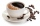They have to prepare a mixture of coffee in packing centres so that 1 kg costs 240 CZK. There are two types of coffee in stock at the price of 220 CZK per 1 kg and 300 CZK per 1 kg. How many kilograms of each species need to be mixed to prepare 50 kg of t
• Mixture of nutsThe mixture of nuts should be prepared from almonds, peanuts and cashew nuts ratio 1: 2: 3 (respectively). The price of almonds is 150 CZK/kg, the price of peanuts is 140 CZK/kg and the price of cashew nuts is 180 CZK/kg. The price of the mixture is deter
• Alcohol mixtureFrom 55% and 80% alcohol, we have to produce 0.2 kg of 60% alcohol. How many of them do we use in the solution?
• MixingIf we mix 5 kg of goods of one kind and 3 kg second one, resulting mixture cost 16.50 EUR/kg. If these quantities are mixed in reverse - first three kilograms and 5 kilograms second cost of mixture is 18.50 EUR/kg. What is the price of one kg of goods ofThe fruit salad is prepared from oranges and bananas. One kilogram of oranges costs 30 CZK, and one kilogram of bananas costs 18 CZK. How many kilograms of bananas need to be added to half a kilogram of oranges to produce a fruit salad worth CZK 21 per 1
• Tea mixture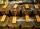Of the two sort of tea at a price of 180 CZK/kg and 240 CZK/kg we make a mixture 12 kg that should be prepared at a price of 200 CZK / kg. How many kilos of each sort of tea will we need to be mixed?
• Apples 2Dried apples contain 15% water. Fresh apples contain 80% water. How many kg of apples we need buy in order to get 3 kg of dried apples?
• SpiritFrom 55% and 80% spirit we would like to produce 0.2 kg of 60% spirit. How many of them we must use in a solution?
• SaltSeawater containing 5% salt. How many kg of freshwater necessary to pour into 40 kg of seawater so that the salinity fell to 2%?
• Fruit juice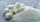Fruit juice contains 37.5% sugar. How many percents of sugar will be in the flavored mineral that we prepare from 100 grams of fruit juice and 1.4 liters of mineral? (1 liter = 1 kg)
• Metal alloy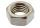What is the ratio of metals in the alloy that is in the 50 tonnes of steel to 30 kg nickel?
• Two teas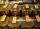A mixture weighing 10 kg was made from two types of tea. The price of tea was 160 CZK/kg and second tea 170 CZK/kg. The price of the mixture is 166 CZK/kg. How many kilograms of each type of tea had to be mixed?
• CandiesThe price for 1 kg of more expensive candies is 125 CZK. The price for 1 kg of cheaper candy is 100 CZK. We mix two different mixtures of candy. A) The first mixture contains 2 kg more expensive and 0.5 kg cheaper candy. Calculate the price for 1 kg of th
• Cork and swimmingIf a person weighs 80 kg, how many kilograms of cork must take a swimming belt to float on water? The density of the human body is 1050kg/m3 and cork 300kg/m3. (Instructions: Let the human body and cork on a mixture with a density of 1000kg/m3. When you m
• MushroomsFresh mushrooms contain 88% water, dried 14% water. How many kilograms of fresh mushrooms should be collected to get 3 kg of dried?
• Three piles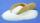100 kg of sugar we divide into three piles. The first pile is small. If we added to the second 2 kg of sugar, it would have 25% more sugar than the first pile. If we add 3 kg of sugar to the third pile it would have 20% more sugar than the 2nd pile. How m